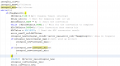# Designing PI controller anti-windup

#### Pedro_Lopes_951

Joined Mar 24, 2020
9
Hello everyone,
I am having some doubts about how to insert an anti-windup on my Microcontroller PIC dsPIC33EV. I designed a PI controller to control the charging of a batterie and I think all expect the Anti-wind up is correct. I will insert a screenshot of the C code I wrote of the Controler and the PI schematic I have done in Matlab so that if anyone knows how can I implement the PI on the microcontroller.#### Attachments

Last edited by a moderator:

#### trebla

Joined Jun 29, 2019
470
Why do you think if something is wrong with the code? How you use PI calculation output? Please insert your code between code tags (in edit window top menu Insert->Code) so people here will be able to see your code better.

#### Pedro_Lopes_951

Joined Mar 24, 2020
9
C:
Kp=1;//Porpotional Gain
Ki=0.1;//Integral Gain
Kw=0.1;//anti-windup Gain
Tsampling=100*10^-6;
error_old=0;
integral_old=0;
integral_max=1;//
integral_min=-1;//
while(1){
if(flagMain==1){
IEC0bits.T1IE = 0;// Disable Timer1 interrupt
Delay_us(10); // Wait for sampling time (10 us)
AD1CON1bits.SAMP = 0; // Start the conversion
while (!AD1CON1bits.DONE); // Wait for the conversion to complete
integral_new=integral_old+Ki*(error_new+error_old)*Tsampling/2;// Area do trapezio
if(integral_new>integral_max){//Anti wind up protect
integral_new=integral_max;//
}
if((integral_new<integral_min)){
integral_new=integral_min;
}
}
PHASE2=  Kp*error_new+integral_new;//Ouput of the controller
integral_old=integral_new;
error_old=error_new;

#### Pedro_Lopes_951

Joined Mar 24, 2020
9
Why do you think if something is wrong with the code? How you use PI calculation output? Please insert your code between code tags (in edit window top menu Insert->Code) so people here will be able to see your code better.
I just don't think the way I am doing the anti-windup is correct. The Phase2 variable is where the output of the controller.
C:
Kp=1;//Porpotional Gain
Ki=0.1;//Integral Gain
Kw=0.1;//anti-windup Gain
Tsampling=100*10^-6;
error_old=0;
integral_old=0;
integral_max=1;//
integral_min=-1;//
while(1){
if(flagMain==1){
IEC0bits.T1IE = 0;// Disable Timer1 interrupt
Delay_us(10); // Wait for sampling time (10 us)
AD1CON1bits.SAMP = 0; // Start the conversion
while (!AD1CON1bits.DONE); // Wait for the conversion to complete
integral_new=integral_old+Ki*(error_new+error_old)*Tsampling/2;// Area do trapezio
if(integral_new>integral_max){//Anti wind up protect
integral_new=integral_max;//
}
if((integral_new<integral_min)){
integral_new=integral_min;
}
}
PHASE2=  Kp*error_new+integral_new;//Ouput of the controller
integral_old=integral_new;
error_old=error_new;

#### trebla

Joined Jun 29, 2019
470
Are you tested how this code works? At first look i suggest add one windup countermeasure: if error is zero make integral_new also zero.

#### crutschow

Joined Mar 14, 2008
28,194
Integrator windup is one of the problems of emulating an analog linear feedback network designed for linear system elements.
You might consider digital Fuzzy Logic loop control, which doesn't use integrators and can also readily handle non-linear system elements.